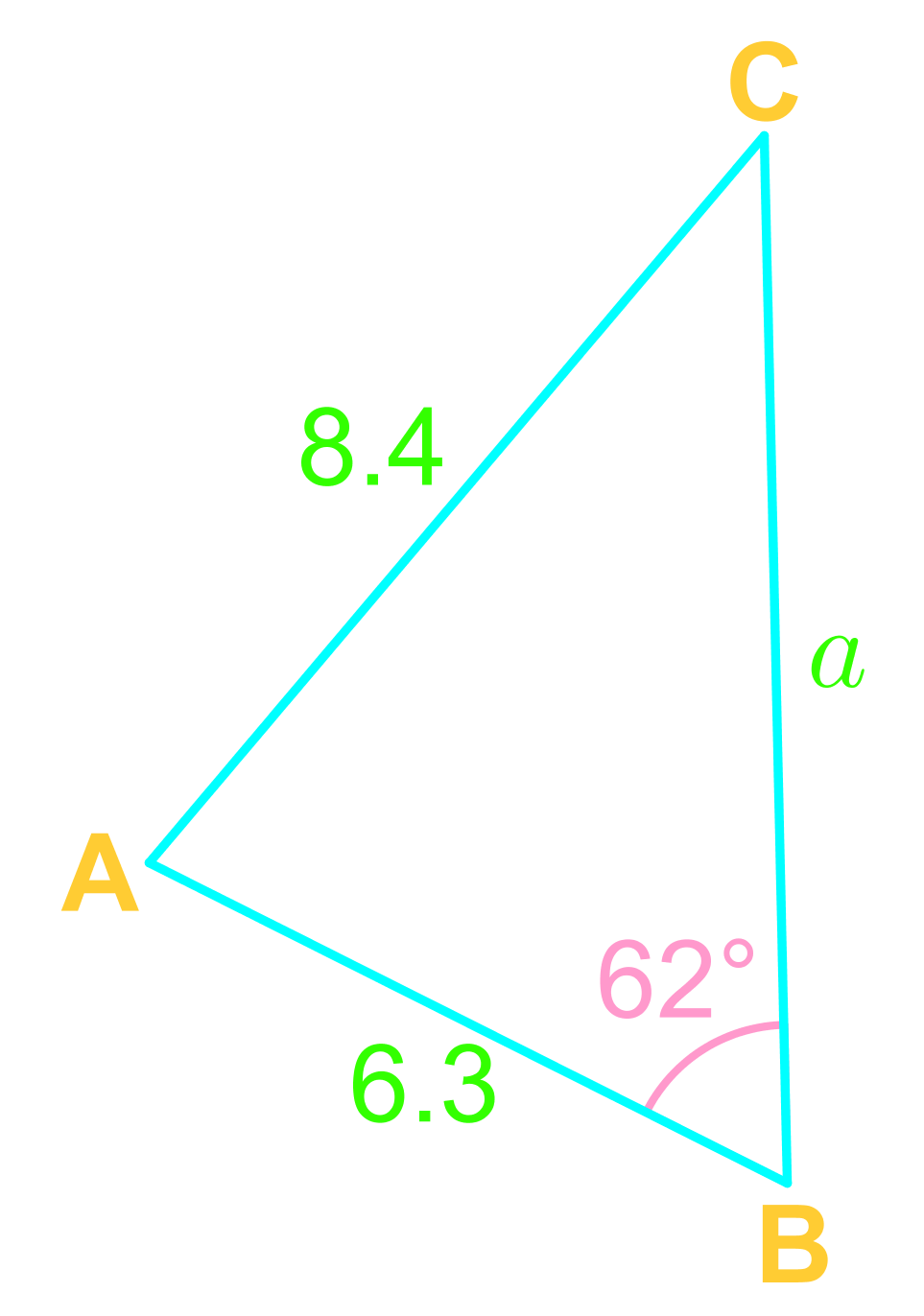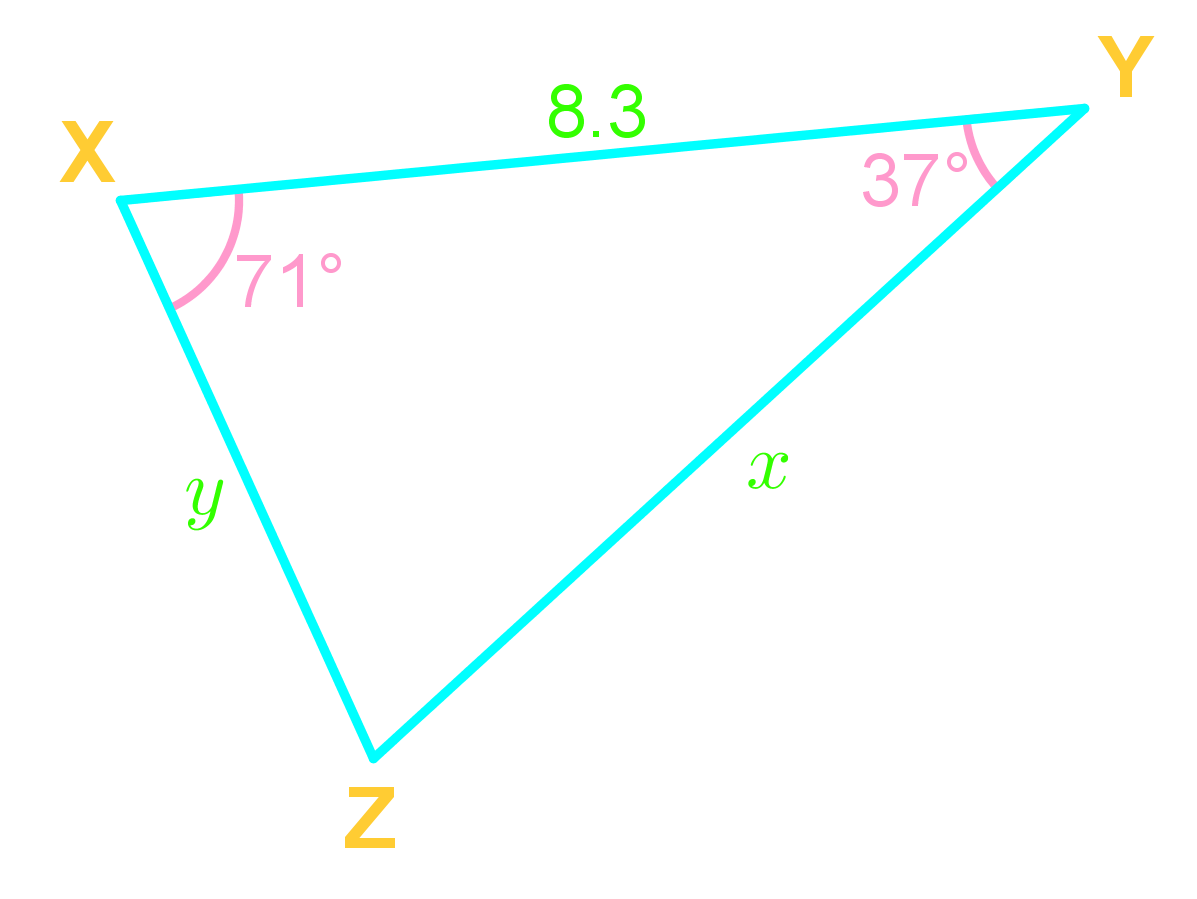Law of sines

Law of sines

In this section, we will learn about the Law of Sines, also known as the Sines Rule. The Law of Sines is a formula that models the relationship between the sides and the angles of any triangle, be it a right-angled triangle, an obtuse triangle, or an acute triangle. In order to use the Law of Sines, we need to satisfy the "one pair, one additional information" condition (i.e. Angle-Angle-Side abbreviated as AAS, and Angle-Side-Angle abbreviated as ASA). We will also explore the concept of the Ambiguous Case of the Law of Sines.

Lessons

Law of Sine
For any $\triangle$ ABC,

$\frac{a}{\sin(A)}$ $=\frac{b}{\sin(B)}$ $=\frac{c}{\sin(C)}$
and,
$\frac{\sin(A)}{a}$ $=\frac{\sin(B)}{b}$ $=\frac{\sin(C)}{c}$

Use the Law of Sine when given a pair!

Ambiguous case
Ambiguous case of the Law of Sine arises when given SSA (side-side-angle)

Step 1) Use the given angle to find the height of the triangle: $h=b \sin (A)$

Step 2) Check if,
$Side\;a$ < $h$,
then no triangles
$Side\;a=h$,
then 1 triangle
$Side\;a$ > $h$,
then 1 triangle
$h$ < $Side\;a$ < $Side\;b$,
then 2 triangles

Step 3) Solve the triangle(s)!
• Introduction

• 1.
Given the following triangle $\triangle ABC$,a)
Solve for$\angle C$

b)
Solve for $a$

• 2.
Solve for side $x$• 3.
Ambiguous case: SSA triangles
In $\triangle DEF$, $DE=21cm$, $\angle$$F=45$°, and $EF=24cm$; find $DF$.